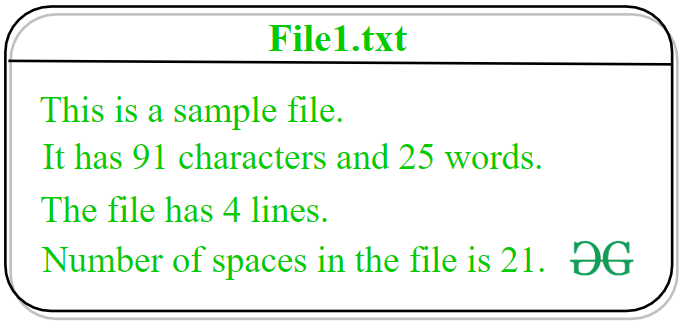Related Articles

# Python – Get number of characters, words, spaces and lines in a file

• Difficulty Level : Easy
• Last Updated : 29 Dec, 2020

Prerequisite: File Handling in Python

Given a text file fname, the task is to count the total number of characters, words, spaces and lines in the file.

As we know, Python provides multiple in-built features and modules for handling files. Let’s discuss different ways to calculate total number of characters, words, spaces and lines in a file using Python.Method #1: Naive approach
In this approach, the idea is to solve the task by developing our own logic. Without using any built-in function of Python, the total number of characters, words, spaces and lines of the file will be calculated.

Below is the implementation of the above approach.

 `# Python implementation to compute``# number of characters, words, spaces``# and lines in a file`` ` `# Function to count number ``# of characters, words, spaces ``# and lines in a file``def` `counter(fname):`` ` `    ``# variable to store total word count``    ``num_words ``=` `0``     ` `    ``# variable to store total line count``    ``num_lines ``=` `0``     ` `    ``# variable to store total character count``    ``num_charc ``=` `0``     ` `    ``# variable to store total space count``    ``num_spaces ``=` `0``     ` `    ``# opening file using with() method``    ``# so that file gets closed ``    ``# after completion of work``    ``with ``open``(fname, ``'r'``) as f:``         ` `        ``# loop to iterate file``        ``# line by line``        ``for` `line ``in` `f:``             ` `            ``# incrementing value of ``            ``# num_lines with each ``            ``# iteration of loop to``            ``# store total line count ``            ``num_lines ``+``=` `1``             ` `            ``# declaring a variable word``            ``# and assigning its value as Y``            ``# because every file is ``            ``# supposed to start with ``            ``# a word or a character``            ``word ``=` `'Y'``             ` `            ``# loop to iterate every``            ``# line letter by letter``            ``for` `letter ``in` `line:``                 ` `                ``# condition to check ``                ``# that the encountered character``                ``# is not white space and a word``                ``if` `(letter !``=` `' '` `and` `word ``=``=` `'Y'``):``                     ` `                    ``# incrementing the word``                    ``# count by 1``                    ``num_words ``+``=` `1``                     ` `                    ``# assigning value N to ``                    ``# variable word because until``                    ``# space will not encounter``                    ``# a word can not be completed``                    ``word ``=` `'N'``                     ` `                ``# condition to check ``                ``# that the encountered character``                ``# is a white space``                ``elif` `(letter ``=``=` `' '``):``                     ` `                    ``# incrementing the space``                    ``# count by 1``                    ``num_spaces ``+``=` `1``                     ` `                    ``# assigning value Y to``                    ``# variable word because after``                    ``# white space a word``                    ``# is supposed to occur``                    ``word ``=` `'Y'``                     ` `                ``# loop to iterate every ``                ``# letter character by ``                ``# character``                ``for` `i ``in` `letter:``                     ` `                    ``# condition to check ``                    ``# that the encountered character ``                    ``# is not  white space and not``                    ``# a newline character``                    ``if``(i !``=``" "` `and` `i !``=``"\n"``):``                         ` `                        ``# incrementing character``                        ``# count by 1``                        ``num_charc ``+``=` `1``                         ` `    ``# printing total word count ``    ``print``(``"Number of words in text file: "``, num_words)``     ` `    ``# printing total line count``    ``print``(``"Number of lines in text file: "``, num_lines)``     ` `    ``# printing total character count``    ``print``(``'Number of characters in text file: '``, num_charc)``     ` `    ``# printing total space count``    ``print``(``'Number of spaces in text file: '``, num_spaces)``     ` `# Driver Code: ``if` `__name__ ``=``=` `'__main__'``: ``    ``fname ``=` `'File1.txt'``    ``try``: ``        ``counter(fname) ``    ``except``: ``        ``print``(``'File not found'``)`

Output:

```Number of words in text file:  25
Number of lines in text file:  4
Number of characters in text file:  91
Number of spaces in text file:  21
```

Method #2: Using some built-in functions and OS module functions
In this approach, the idea is to use the `os.linesep()` method of OS module to separate the lines on the current platform. When the interpreter’s scanner encounter `os.linesep` it replaces it with `\n` character. After that `strip()` and `split()` functions will be used to carry out the task.
Get more idea about `strip()` and `split()` functions.

Below is the implementation of the above approach.

 `# Python implementation to compute``# number of characters, words, spaces``# and lines in a file`` ` `# importing os module``import` `os`` ` `# Function to count number ``# of characters, words, spaces ``# and lines in a file``def` `counter(fname):``     ` `    ``# variable to store total word count``    ``num_words ``=` `0``     ` `    ``# variable to store total line count``    ``num_lines ``=` `0``     ` `    ``# variable to store total character count``    ``num_charc ``=` `0``     ` `    ``# variable to store total space count``    ``num_spaces ``=` `0``     ` `    ``# opening file using with() method``    ``# so that file gets closed ``    ``# after completion of work``    ``with ``open``(fname, ``'r'``) as f:``         ` `        ``# loop to iterate file``        ``# line by line``        ``for` `line ``in` `f:``             ` `            ``# separating a line ``            ``# from \n character ``            ``# and storing again in line ``            ``# variable for further operations``            ``line ``=` `line.strip(os.linesep)``             ` `            ``# splitting the line ``            ``# to make a list of``            ``# all the words present``            ``# in that line and storing``            ``# that list in``            ``# wordlist variable``            ``wordslist ``=` `line.split()``             ` `            ``# incrementing value of ``            ``# num_lines with each ``            ``# iteration of loop to``            ``# store total line count``            ``num_lines ``=` `num_lines ``+` `1``             ` `            ``# incrementing value of ``            ``# num_words by the ``            ``# number of items in the``            ``# list wordlist``            ``num_words ``=` `num_words ``+` `len``(wordslist)``             ` `            ``# incrementing value of ``            ``# num_charc by 1 whenever``            ``# value of variable c is other ``            ``# than white space in the separated line``            ``num_charc ``=` `num_charc ``+` `sum``(``1` `for` `c ``in` `line ``                          ``if` `c ``not` `in` `(os.linesep, ``' '``))``             ` `            ``# incrementing value of ``            ``# num_spaces by 1 whenever``            ``# value of variable s is ``            ``# white space in the separated line``            ``num_spaces ``=` `num_spaces ``+` `sum``(``1` `for` `s ``in` `line ``                                ``if` `s ``in` `(os.linesep, ``' '``))``     ` `    ``# printing total word count``    ``print``(``"Number of words in text file: "``, num_words)``     ` `    ``# printing total line count``    ``print``(``"Number of lines in text file: "``, num_lines)``     ` `    ``# printing total character count``    ``print``(``"Number of characters in text file: "``, num_charc)``     ` `    ``# printing total space count``    ``print``(``"Number of spaces in text file: "``, num_spaces)`` ` `# Driver Code: ``if` `__name__ ``=``=` `'__main__'``: ``    ``fname ``=` `'File1.txt'``    ``try``: ``        ``counter(fname) ``    ``except``: ``        ``print``(``'File not found'``)`

Output:

```Number of words in text file:  25
Number of lines in text file:  4
Number of characters in text file:  91
Number of spaces in text file:  21
```

Attention geek! Strengthen your foundations with the Python Programming Foundation Course and learn the basics.

To begin with, your interview preparations Enhance your Data Structures concepts with the Python DS Course. And to begin with your Machine Learning Journey, join the Machine Learning – Basic Level Course

My Personal Notes arrow_drop_up